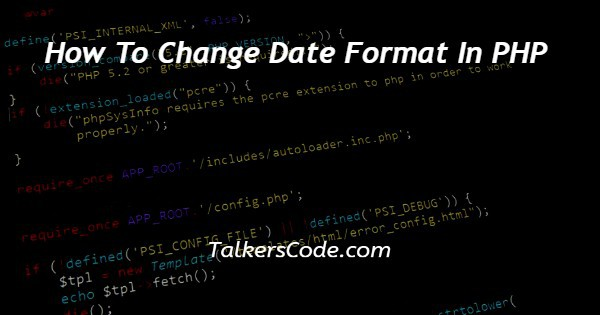# How To Change Date Format In PHP

Last Updated : Jan 1, 2023In this tutorial we will show you the solution of how to change date format in PHP, sometimes in PHP, we need to change the current date format.

For example, if the current date format is yyyy-mm-dd and we want to get date in dd-mm-yyyy.

Hence, here we need something which helps us to change date format.

In this tutorial, we will learn about how to change date format in php.

## Step By Step Guide On How To Change Date Format In PHP :-

Here, here below we are going to show you how to convert date format.

One more thing is sometimes the time is displayed in 24-hour format and we want 12-hour format. We will also learn about this topic in this article. Below is code given in php and lets start understand that code.

```<?php
function stringConversion (\$date)
{
// conversion of date and time to sec
\$sec = strtotime(\$date);
// conversion of date and time to specified format
\$date = date("Y-m-d H:i", \$sec);
// append seconds to the date and time
\$date = \$date . ":00";
// print required date and time
echo \$date;
}
\$date = "07/11/2002 09:18 AM";
stringConversion(\$date);
?>```
1. Now, let us understand the codes step by step.
2. Here, first of all as you see in the code that we create basic structure of php code by opening and closing main php tags that are <?php and ?>.
3. In next step, under main php tags we create a function named stringConversion with one parameter.
4. After that strtotime. This help us to change the date format. When user or someone enter the date then if brings that date and convert the date and time into seconds. The seconds are then stored into a variable named \$sec. The syntax of strtotime function is strtotime(parameter).
5. Now, in next step we have to change the second that are stored in \$sec to required format which we want. To change seconds into date and time, we use here date function. Inside date function we have to specify the format with or without time. The general syntax of date function is given as: date(“required_fomat_here”, stored_seconds_variable).
6. You can see the example above in our code. Now, the next line is optional. We use this because we want to show seconds also. That we add manually there.
7. Now, at last step we use echo with \$date. Here, \$date is the variable in which we store the new date with required format. Whereas echo is used to print the date.
8. After function, we just store a date in \$date variable with time and call the function with required parameter. The function itself manipulate the real date and change it to required format and also print it.
9. We hope that you understand this article properly. Now, here is one assignment for you in which you have to change different date formats with or without time with the help of this article.

## Conclusion :-

At last in conclusion, here we can say that with the help of this article we are able to change date format in php.

I hope this tutorial on how to change date format in PHP helps you and the steps and method mentioned above are easy to follow and implement.